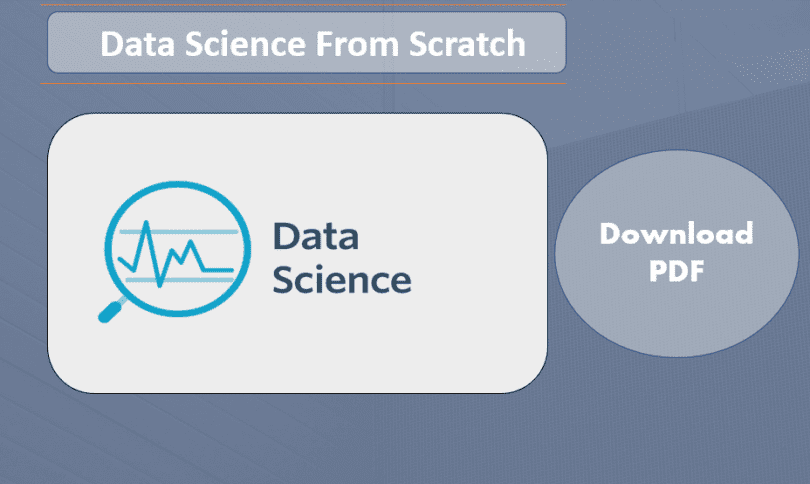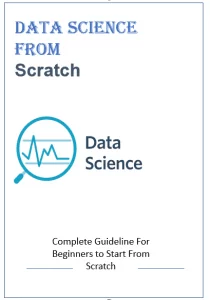# Data Science From Scratch Learning PDF NotesDownload data science from scratch learning PDF notes free with the help of these points you will be able to learn about data science from Scratch (beginning) .As You Know Data Science is Hot and Growing Field in 21 Century .

As you have seen There are lots of data science libraries, frameworks, modules, and toolkits that efficiently implement the most common data science algorithms and techniques.

Whenever you  become a data scientist, you need to Learn  NumPy, with scikit-learn, with pandas, and with a panoply of other libraries.

 Notes Data Science From Scratch Format word Language English Size 16MB Addition To help you to solve data analysis problems For Beginners

You will Learn These Stuff

Get in touch basics of programming with Python

Some real time Python code Examples with Different Python libraries like NumPy etc.

Get in touch basic Statistics and Mathematics. as you Know Math and Statistics are Heart of Data Science. if we are going to start learning Data Science we need to know about Math and Statistics.

Get in touch Python for Data Analysis.

Then start  Machine Learning from Scratch with Different Principle .

Discuss some good real time Practice with projects.

These Topic are Covered very well in these notes. even simple Beginner person can start learning from these notes we are sharing this PDF Free of cost with you.